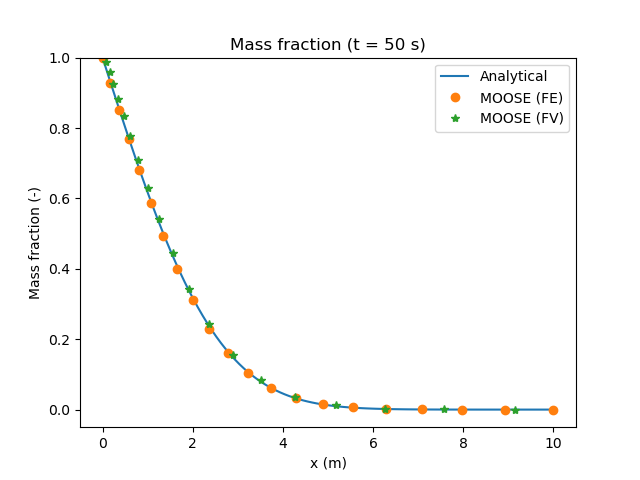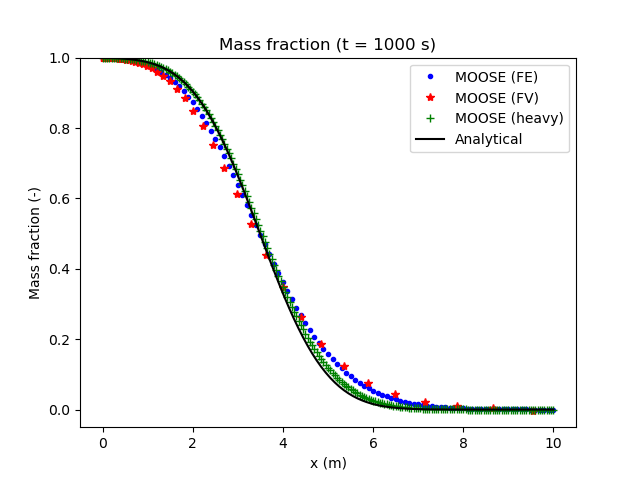# Diffusion and hydrodynamic dispersion Tests

## Diffusion

The results of PorousFlow are compared with the classical diffusion profile for a simple 1D model with mass diffusion. In this example, the left end of a 1D mesh is held at a constant mass fraction of 1, while the right hand end is prescribed a zero mass fraction boundary condition. No advection takes place, so mass transfer is by diffusion only. The input file:

# Test diffusive part of PorousFlowDispersiveFlux kernel by setting dispersion
# coefficients to zero. Pressure is held constant over the mesh, and gravity is
# set to zero so that no advective transport of mass takes place.
# Mass fraction is set to 1 on the left hand side and 0 on the right hand side.

[Mesh]
type = GeneratedMesh
dim = 1
nx = 20
xmax = 10
bias_x = 1.1
[]

[GlobalParams]
PorousFlowDictator = andy_heheheh
[]

[Variables]
[./pp]
[../]
[./massfrac0]
[../]
[]

[ICs]
[./pp]
type = ConstantIC
variable = pp
value = 1e5
[../]
[./massfrac0]
type = ConstantIC
variable = massfrac0
value = 0
[../]
[]

[BCs]
[./left]
type = PresetBC
value = 1
variable = massfrac0
boundary = left
[../]
[./right]
type = PresetBC
value = 0
variable = massfrac0
boundary = right
[../]
[./pright]
type = PresetBC
variable = pp
boundary = right
value = 1e5
[../]
[./pleft]
type = PresetBC
variable = pp
boundary = left
value = 1e5
[../]
[]

[Kernels]
[./diff0]
type = PorousFlowDispersiveFlux
fluid_component = 0
variable = massfrac0
disp_trans = 0
disp_long = 0
gravity = '0 0 0'
[../]
[./diff1]
type = PorousFlowDispersiveFlux
fluid_component = 1
variable = pp
disp_trans = 0
disp_long = 0
gravity = '0 0 0'
[../]
[]

[Modules]
[./FluidProperties]
[./the_simple_fluid]
type = SimpleFluidProperties
thermal_expansion = 0.0
bulk_modulus = 1E7
viscosity = 0.001
density0 = 1000.0
[../]
[../]
[]

[PorousFlowUnsaturated]
porepressure = pp
gravity = '0 0 0'
fp = the_simple_fluid
dictator_name = andy_heheheh
relative_permeability_type = Corey
relative_permeability_exponent = 0.0
mass_fraction_vars = massfrac0
[]

[Materials]
[./poro]
type = PorousFlowPorosityConst
porosity = 0.3
[../]
[./diff]
type = PorousFlowDiffusivityConst
diffusion_coeff = '1 1'
tortuosity = 0.1
[../]
[./permeability]
type = PorousFlowPermeabilityConst
permeability = '1e-9 0 0 0 1e-9 0 0 0 1e-9'
[../]
[]

[Preconditioning]
[./smp]
type = SMP
full = true
petsc_options_iname = '-ksp_type -pc_type -sub_pc_type -sub_pc_factor_shift_type -pc_asm_overlap'
petsc_options_value = 'gmres      asm      lu           NONZERO                   2             '
[../]
[]

[Executioner]
type = Transient
solve_type = NEWTON
dt = 1
end_time = 20
[]

[VectorPostprocessors]
[./xmass]
type = NodalValueSampler
sort_by = id
variable = massfrac0
[../]
[]

[Outputs]
[./out]
type = CSV
execute_on = final
[../]
[]

(modules/porous_flow/test/tests/dispersion/diff01_action.i)

This concentration profile has a well-known similarity solution given by (1) where is the complentary error function, and is the similarity solution, is distance, is time, and is the diffusion coefficient.

The comparison between PorousFlow and this analytical solution is presented in Figure 1, where we observe a very good agreement between the two solutions.Figure 1: Mass fraction profile from diffusion only.

## Hydrodynamic dispersion

The PorousFlow results are compared to known analytical solutions for simple problems in order to verify that the MOOSE implementation is working properly. For a simple 1D model with no diffusion and constant velocity , an analytical solution for the mass fraction profile is given by (Javandel et al., 1984) (2) where all parameters have been previously defined.

The input file:

# Test dispersive part of PorousFlowDispersiveFlux kernel by setting diffusion
# coefficients to zero. A pressure gradient is applied over the mesh to give a
# uniform velocity. Gravity is set to zero.
# Mass fraction is set to 1 on the left hand side and 0 on the right hand side.

[Mesh]
type = GeneratedMesh
dim = 1
nx = 200
xmax = 10
[]

[GlobalParams]
PorousFlowDictator = dictator
gravity = '0 0 0'
compute_enthalpy = false
compute_internal_energy = false
[]

[Variables]
[./pp]
[../]
[./massfrac0]
[../]
[]

[AuxVariables]
[./velocity]
family = MONOMIAL
order = FIRST
[../]
[]

[AuxKernels]
[./velocity]
type = PorousFlowDarcyVelocityComponent
variable = velocity
component = x
[../]
[]

[ICs]
[./pp]
type = FunctionIC
variable = pp
function = pic
[../]
[./massfrac0]
type = ConstantIC
variable = massfrac0
value = 0
[../]
[]

[Functions]
[./pic]
type = ParsedFunction
value = 1.1e5-x*1e3
[../]
[]

[BCs]
[./xleft]
type = PresetBC
value = 1
variable = massfrac0
boundary = left
[../]
[./xright]
type = PresetBC
value = 0
variable = massfrac0
boundary = right
[../]
[./pright]
type = PresetBC
variable = pp
boundary = right
value = 1e5
[../]
[./pleft]
type = PresetBC
variable = pp
boundary = left
value = 1.1e5
[../]
[]

[Kernels]
[./mass0]
type = PorousFlowMassTimeDerivative
fluid_component = 0
variable = pp
[../]
[./adv0]
type = PorousFlowAdvectiveFlux
fluid_component = 0
variable = pp
[../]
[./diff0]
type = PorousFlowDispersiveFlux
variable = pp
disp_trans = 0
disp_long = 0.2
[../]
[./mass1]
type = PorousFlowMassTimeDerivative
fluid_component = 1
variable = massfrac0
[../]
[./adv1]
type = PorousFlowAdvectiveFlux
fluid_component = 1
variable = massfrac0
[../]
[./diff1]
type = PorousFlowDispersiveFlux
fluid_component = 1
variable = massfrac0
disp_trans = 0
disp_long = 0.2
[../]
[]

[UserObjects]
[./dictator]
type = PorousFlowDictator
porous_flow_vars = 'pp massfrac0'
number_fluid_phases = 1
number_fluid_components = 2
[../]
[]

[Modules]
[./FluidProperties]
[./simple_fluid]
type = SimpleFluidProperties
bulk_modulus = 1e9
density0 = 1000
viscosity = 0.001
thermal_expansion = 0
[../]
[../]
[]

[Materials]
[./temperature]
type = PorousFlowTemperature
[../]
[./ppss]
type = PorousFlow1PhaseFullySaturated
porepressure = pp
[../]
[./massfrac]
type = PorousFlowMassFraction
mass_fraction_vars = massfrac0
[../]
[./simple_fluid]
type = PorousFlowSingleComponentFluid
fp = simple_fluid
phase = 0
[../]
[./poro]
type = PorousFlowPorosityConst
porosity = 0.3
[../]
[./diff]
type = PorousFlowDiffusivityConst
diffusion_coeff = '0 0'
tortuosity = 0.1
[../]
[./relp]
type = PorousFlowRelativePermeabilityConst
phase = 0
[../]
[./permeability]
type = PorousFlowPermeabilityConst
permeability = '1e-9 0 0 0 1e-9 0 0 0 1e-9'
[../]
[]

[Preconditioning]
[./smp]
type = SMP
full = true
petsc_options_iname = '-ksp_type -pc_type -sub_pc_type -sub_pc_factor_shift_type -pc_asm_overlap'
petsc_options_value = 'gmres      asm      lu           NONZERO                   2             '
[../]
[]

[Executioner]
type = Transient
solve_type = NEWTON
end_time = 1e3
dtmax = 10
[./TimeStepper]
type = IterationAdaptiveDT
growth_factor = 1.5
cutback_factor = 0.5
dt = 1
[../]
[]

[VectorPostprocessors]
[./xmass]
type = NodalValueSampler
sort_by = id
variable = massfrac0
[../]
[]

[Outputs]
[./out]
type = CSV
execute_on = final
[../]
[]

(modules/porous_flow/test/tests/dispersion/disp01_heavy.i)

The comparison between the PorousFlow and the analytical formula is presented in Figure 2. For the non-heavy case, the MOOSE results do not coincide with the analytical solution near the top and bottom of the concentration front due to numerical dispersion. If the number of elements in the mesh is increased and the time step size is reduced (the "heavy" case), numerical dispersion is reduced and a much closer fit to the analytical solution is obtained.Figure 2: Mass fraction profile from hydrodynamic dispersion only.

1. I. Javandel, C. Doughty, and C. F. Tsang. Groundwater Transport, Handbook of Mathematical Models. AGU, 1984.[BibTeX]